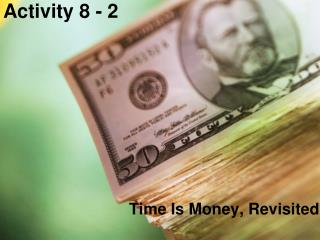DownloadDownload PresentationActivity 8 - 2

# Activity 8 - 2

Télécharger la présentation## Activity 8 - 2

- - - - - - - - - - - - - - - - - - - - - - - - - - - E N D - - - - - - - - - - - - - - - - - - - - - - - - - - -
##### Presentation Transcript

1. Activity 8 - 2 Time Is Money, Revisited

2. Objectives • Distinguish between simple and compound interest • Apply the compound interest formula to determine the future value of a lump-sum investment earning compound interest • Determine the future value using technology • Determine the effective interest rate • Apply the present value formula in a given situation involving compound interest • Determine the present value using technology

3. Vocabulary • Simple Interest – interest paid on principal amount only • Compound Interest – interest paid on interest as well as principal amount • Effective Annual Yield – is also called the APY • Annual Percentage Yield (APY) – is the true rate of return on an investment • Present Value – amount that must be invested now at compound interest to reach a given future value • Future Value – amount that an investment will be worth at a future time if invested at compound interest

4. Activity Suppose \$10,000 is deposited in a bank at 6.5% annual interest. What is the interest earned after one year? Suppose you left the money in the account for 10 years. Can the total amount of interest be calculated by multiplying your answer above by 10? What type of interest would this be? 10,000  0.065 = \$650 Maybe: 10  \$650 = \$6500 Only if it is simple interest.

5. Compound Interest Formula If interest is compounded, then the current balance is given by the formula A = P(1 + r/n)nt where A is the current balance or compound amount P is the principal (original amount deposited) r is the annual interest rate (in decimal form) n is the number of times per year that the interest is compounded t is the time in years that money has been invested

6. Using our Calculator We have an application on our calculator called Finances. Under this application we have a program called TVM Solver that will solve many of the financial problems that we will deal with in this chapter. Press the APPS key, select Finances and ENTER The TVM Solver is the first option under CALC. Hit ENTER and see the list of variables that we need to find values for and which ones we can solve for.

7. TI TVM Solver • N = total number of compounding periods • I% = r, where r% is the annual interest rate • PV = present value (amount invested or principal) • PMT = payment • FV = future payment • P/Y = number of payments per year • C/Y = number of compounding periods per year • PMT/END = payment due at the end of the period • PMT/BEGIN = payment due at the beginning of the period • Use ALPHA Enter on a line to solve for that item

8. Compound Interest Examples • Suppose you deposit \$10,000 in an account that has a 6.5% annual interest rate and whose interest is compounded annually. How much do you have after 5 years? • If compounded quarterly? • If compounded monthly? N=5, I%=6.5, PV=10000, P/Y=C/Y=1, PMT:END FV = \$13,700.87 N=5*4, I%=6.5, PV=10000, P/Y=C/Y=4, PMT:END FV = \$13,804.20 N=5*12, I%=6.5, PV=10000, P/Y=C/Y=12, PMT:END FV = \$13,828.17

9. Effective Annual Yield The effective annual yield of an investment depends on both the interest rate and how often it is being compounded. Most financial institutions refer to the effective yield as the annual percentage yield or APY. Using your calculator: Press APPS, Finance, arrow down to C:Eff( and ENTER. Type in the rate followed by the number of compounding periods and “)”. Press ENTER and the effective annual rate will appear on the screen.

10. Effective Annual Yield Example Determine the annual percentage yield (APY) on an investment of \$1200 at 10% annual interest rate compounded monthly. Using Finances App select C:Eff( type in 10, 12) APY = 10.47% Note invested amount does not figure in!

11. Present Value The amount to be invested is called the present value and in previous problems was known and you needed to determine the future value of the investment. In a present value problem, the future value is known (you need a set amount at a future date) and you must determine how much you must initially deposit to get this amount in the future. If we solve the compound interest formula for P, we get FV PV = ---------------- (1 + r/n)nt These problems can also be solved using TVM Solver

12. Present Value Example A local bank is offering a 60-month CD at a rate of 5.7% APY compounded monthly. How much must be invested now in the CD to accumulate \$6000 at the end of 5 years? Using TVM Solver with: N=60, I%=5.7, FV=6000, P/Y=C/Y=12, PMT:END PV = \$4515.13

13. Summary and Homework • Summary • Compound interest is interest paid on interest as well as the principal • Compound amount (aka future value) is the total amount of investment • The Future value can be calculated using TVM Solver on TI-83 Plus • Effective interest rate is the true rate of return on an investment • Present value is amount that must be invested now to reach a given future value • Homework • page 922-923; problems 1, 3, 4, 7, 9, 10